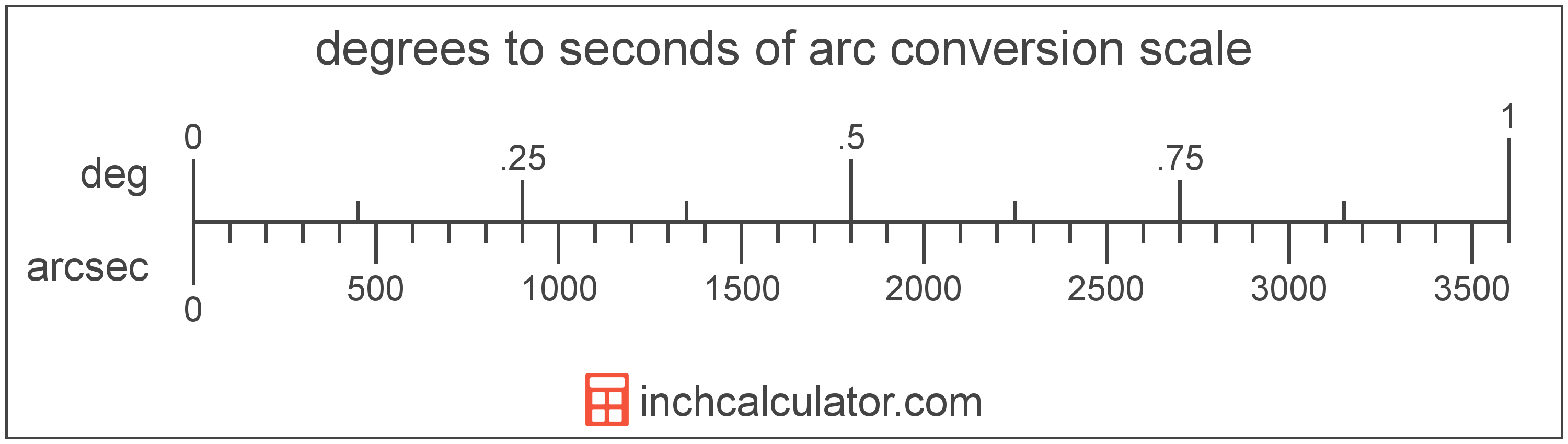# Degrees to Seconds of Arc Converter

Enter the angle in degrees below to get the value converted to seconds of arc.

Results in Seconds of Arc:1° = 3,600 arcsec

Do you want to convert seconds of arc to degrees?

## How to Convert Degrees to Seconds of Arc

To convert a measurement in degrees to a measurement in seconds of arc, multiply the angle by the following conversion ratio: 3,600 seconds of arc/degree.

Since one degree is equal to 3,600 seconds of arc, you can use this simple formula to convert:

seconds of arc = degrees × 3,600

The angle in seconds of arc is equal to the angle in degrees multiplied by 3,600.

For example, here's how to convert 5 degrees to seconds of arc using the formula above.
seconds of arc = (5° × 3,600) = 18,000"### How Many Seconds of Arc Are in a Degree?

There are 3,600 seconds of arc in a degree, which is why we use this value in the formula above.

1° = 3,600"

### Uses for Degrees, Minutes, & Seconds

For measurements in cartography and astronomy, degrees are often expressed in decimal form or degrees, minutes, and seconds (sometimes called DMS). Minutes and seconds are used to express portions of angle smaller than one degree.

You can use our decimal to DMS calculator to convert an angle to degrees, minutes, & seconds form.

## What Is a Degree?

A degree is a measure of angle equal to 1/360th of a revolution, or circle. The number 360 has 24 divisors, making it a fairly easy number to work with. There are also 360 days in the Persian calendar year, and many theorize that early astronomers used 1 degree per day.

The degree is an SI accepted unit for angle for use with the metric system. A degree is sometimes also referred to as a degree of arc, arc degree, or arcdegree. Degrees can be abbreviated as °, and are also sometimes abbreviated as deg. For example, 1 degree can be written as 1° or 1 deg.

Degrees can also be expressed using arcminutes and arcseconds as an alternative to using the decimal form. Arcminutes and arcseconds are expressed using the prime (′) and double-prime (″) characters, respectively, although a single-quote and double-quote are often used for convenience.

One arcminute is equal to 1/60th of a degree, and one arcsecond is equal to 1/60th of an arcminute.

Protractors are commonly used to measure angles in degrees. They are semi-circle or full-circle devices with degree markings allowing a user to measure an angle in degrees. Learn more about how to use a protractor or download a printable protractor.

## What Is a Second of Arc?

The second of arc is a unit of angle equal to 1/60th of one minute of arc or 1/3,600 of one degree.

A second of arc is sometimes also referred to as an arc second or arcsecond. Seconds of arc can be abbreviated as arcsec, and are also sometimes abbreviated as asec. For example, 1 second of arc can be written as 1 arcsec or 1 asec.

The second of arc is most commonly expressed using a double prime (″), though a double quote is often used as well. For example, 1 second of arc is most often written as 1″.

## Degree to Second of Arc Conversion Table

Table showing various degree measurements converted to seconds of arc.
Degrees Seconds Of Arc
0.001° 3.6"
0.002° 7.2"
0.003° 10.8"
0.004° 14.4"
0.005° 18"
0.006° 21.6"
0.007° 25.2"
0.008° 28.8"
0.009° 32.4"
0.01° 36"
0.02° 72"
0.03° 108"
0.04° 144"
0.05° 180"
0.06° 216"
0.07° 252"
0.08° 288"
0.09° 324"
0.1° 360"
0.2° 720"
0.3° 1,080"
0.4° 1,440"
0.5° 1,800"
0.6° 2,160"
0.7° 2,520"
0.8° 2,880"
0.9° 3,240"
3,600"

## References

1. Collins Dictionary, Definition of 'degree', https://www.collinsdictionary.com/us/dictionary/english/degree